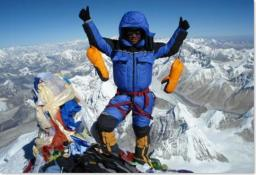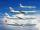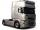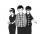# Journey

The road from A to B measures 11.5 km. Firstly up the hill, then by level plane and then downhill. Tourist goes uphill at 3 km/h, on the plane 4 km/h and downhill 5 km/h. From point A to B went 2h 54 min back 3h 6 min. How long is the segment of level plane?

Result

y =  3 km

#### Solution:

x+y+z=11.5
x/3+y/4+z/5 = 2+54/60
x/5+y/4+z/3 = 3+6/60

x+y+z = 11.5
20x+15y+12z = 174
12x+15y+20z = 186

x = 3
y = 4
z = 92 = 4.5

Calculated by our linear equations calculator.Our examples were largely sent or created by pupils and students themselves. Therefore, we would be pleased if you could send us any errors you found, spelling mistakes, or rephasing the example. Thank you!

Leave us a comment of this math problem and its solution (i.e. if it is still somewhat unclear...):Be the first to comment!Tips to related online calculators
Do you have a linear equation or system of equations and looking for its solution? Or do you have quadratic equation?
Do you want to convert velocity (speed) units?
Do you want to convert time units like minutes to seconds?

## Next similar math problems:

1. Theorem proveWe want to prove the sentence: If the natural number n is divisible by six, then n is divisible by three. From what assumption we started?
2. AircraftsAbove the town hall tower flew the plane with constant speed 592 km/h and 15 minutes later the second plane at speed of 675 km/h. How long and how far from the town hall will be aircrafts caught up?
3. Two portsFrom port A on the river, the steamer started at an average speed of 12 km/h towards port B. Two hours later, another steamer departed from A at an average speed of 20 km/h. Both ships arrived in B at the same time. What is the distance between ports A and
4. Truck PRG-BATruck driving on highway from Prague to Bratislava at average speed of 72 km/h. At the moment when the truck passes 54 kilometer from Prague passenger car departs from Prague which travel to Bratislava at speed 90 km/h. When and where (at waht highway ki
5. Boxes200 boxes have been straightened in three rows. The first was 13 more than in the second, and in the second was one fifth more than in the third one. How many boxes are in each row?
6. Father and sonWhen I was 11, my father was 35 years old. Today, my father has three times more years than me. How old is she?
7. ThreesomeDana, Dalibor and Michael have a combined 57 years. Dana is five years older than Dalibor, but Dana is five years younger than Michael. Determine how old is Dana, Dalibor and Michael.
8. Three figures - numbersThe sum of three numbers, if each is 10% larger than the previous one, is 662. Determine the figures.
9. The largerThe larger of two numbers is nine more than four times the smaller number. The sum of the two numbers is fifty-nine. Find the two numbers.
10. Six yearsIn six years Jan will be twice as old as he was six years ago. How old is he?
11. BlackberriesDaniel, Jolana and Stano collected together 34 blackberries. Daniel collected 8 blackberries more than Jolana, Jolana 4 more than Stano. Determine the number blackberries each collected .
12. PearsAndrew, Lenka and Rasto have together 232 pears. Lenka has 28 more than Rasto and Rasto pears have 96 more than Andrew. Determine how much each of them has pears.
13. Football seasonDalibor and Adam together scored 97 goals in the season. Adam scored 9 goals more than Dalibor. How many goals scored each?
14. Lee isLee is 8 years more than twice Park's age, 4 years ago, Lee was three times as old. How old was Lee 4 years ago?
15. SummerjobThree students participated in the summerjob. Altogether they earn 1780, -. Peter got a third less than John and Paul got 100,- more than Peter. How much got every one of them?
16. LegsCancer has 5 pairs of legs. The insect has 6 legs. 60 animals have a total of 500 legs. How much more are cancers than insects?
17. Substitutionsolve equations by substitution: x+y= 11 y=5x-25